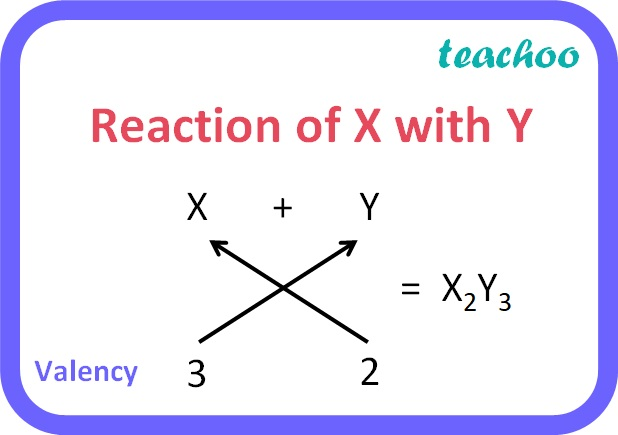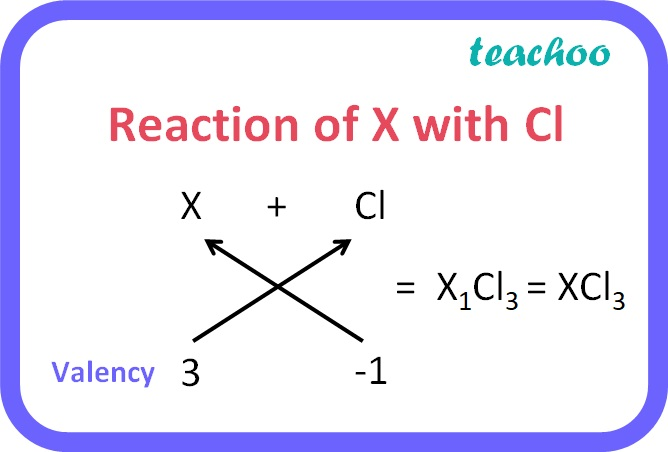Past Year - 3 Mark Questions

Class 10
Chapter 5 Class 10 - Periodic Classification of Elements

## An element ‘X’ belong to 3rd period and group13 of the Modern Periodic Table. (a) Determine the valence electrons and the valency of ‘X’. (b) Molecular formula of the compound formed when ‘X’ reacts with an element ‘Y’(atomic number = 8). (c) Write the name and formula of the compound formed when ‘X’ combines with chlorine.

1. Group Number = 10 + Number of electrons in outermost shell(valence electrons)

So, Number of valence electrons  = 13 - 10 = 3

Therefore, Valency = 3.

2. Atomic number of Y = 8

Electronic configuration of Y: 2 , 6

It has a tendency to gain 2 electrons to become stable i.e. its valency is 2.

Valency of X = 3

Valency of Y = 2The compound they will form is X 2 Y 3 .

3. Chlorine has a valency of -1

X has a valency of 3.The compound they will form is XCl 3 .

Learn in your speed, with individual attention - Teachoo Maths 1-on-1 Class